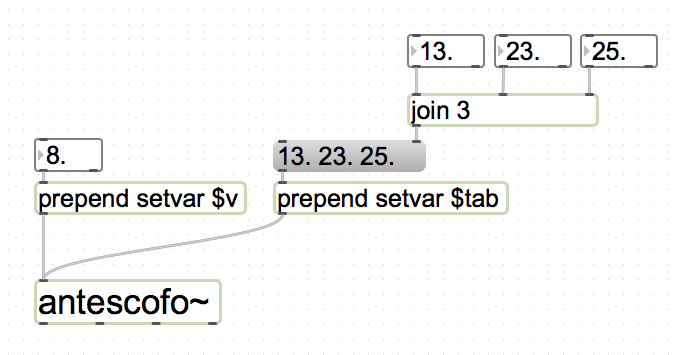## Assignments¶

The assignment of a variable by the value of an expression is an atomic action:

          let $v := exp  Notice the assignation symbol is :=. The operators = and == denote the equality predicate. The keyword let is optional but more clearly distinguishes between the delay and the assigned variable: $d $x := 1 ; is equivalent to$d let $x := 1  In the previous example, the delay is specified by an expression: the variable $d, and the let outlines that the assigned variable is $x and not $d.

A variable has a value before its first assignment: the Undefined value.

Expressions exp in the right hand side of := are described in chapter Expressions.

### Anonymous Assignment¶

The identifier of the assigned variable in the left hand side can be replaced by an underscore _ which is useful to spare a variable when the result of the expression in the right hand side is not needed. This is the case if the expression is evaluated for its side-effects, like dumping values in a file. This action

          _ := exp


simply evaluates the right hand side and discards the result.

Antescofo variables can be assigned from outside Antescofo, using the message setvar in Max or PureData, or an OSC message, see below.

### Assignment to Vector Elements and to Scoped Variables¶

The left hand side of is not restricted to variables. As a matter of fact, there are three kinds of assignments:

1. Assignment to a variable
        let $x := exp  2. the assignment of an element in a tab:  let e[i₁, i₂, ...] := exp  where e is an expression that evaluates to a tab and i₁ , i₂, ..., evaluate to integers (see sect. [mutating a tab element]) 3. the assignment of a local variable in an *exec*:  let e.$x := exp

where e is an expression that evaluates to an *exec* see [Exec] and [outsideScope].

The keyword let is mandatory when expressione is more complex than a variable, i.e. in the last two kinds of assignment.

### Assignment Operators¶

Four assignment operators can be used to abbreviate the expression in the right hand side of an assignment:

        a += e     /* is equivalent to */     a := a + e
a -= e     /* is equivalent to */     a := a - e
a *= e     /* is equivalent to */     a := a * e
a /= e     /* is equivalent to */     a := a / e


The form a in the left hand side can be any form found in the l.h.s. of a simple non-anonymous assignment (e.g., a reference to a variable or the reference to a vector element).

Assignment operators are just notational conveniences and are internally expanded in the form on the right. So, there will be two evaluations of the expression a. For instance:

         $T := TAB[]$cpt_f := 0
@fun_def @f()
{
$cpt_f += 1 return$T
}

let @f() += 10
@assert 10 == $T @assert 2 ==$cpt_f


### Activities Triggered by Assignments¶

The assignment of a value to a variable may trigger some activities:

• the evaluation of a whenever that depends on this variable;

• the reevaluation of the delays that depend on a relative tempo that depend on this variable;

• the reevaluation of the synchroniztion strategies that depend on this variable.

As mentioned in section Delays, the expression specifying a delay is evaluated only once, when the delay is started. It is not re-evaluated after that, even if the variable in the expression are assigned to new values. However, if the delay is expressed in relative time, its conversion in physical time is dynamically adjusted when the corresponding tempo changes.

### External Assignments¶

A global variable may be assigned “from outside Antescofo” in two ways:

1. using the message setvar to the Antescofo object in Max or PureData,

2. using an OSC message.

Section OSCreceive describes the assignment of variables upon the reception of an OSC message.

A simple patch using the setvar message is pictured below. The message takes as its first argument the name of the Antescofo variable to assign.

If there is only a second argument, this argument becomes the value of the variable. Max/PD integers, floats and stringss are handled. If there are several remaining arguments, these arguments are put in a tab (see and the the variable is assigned with this tab value.

External assignments trigger the whenever that may watch the externally assigned variables, cf. whenever. For example, with the patch pictured below, the program:

          whenever ($tab) { print "I just received the vector "$tab
}


will write

      I just received the vector 13 23 25


on the console when the prepend setvar …is activated.### Unassignable variables¶

System variables and special variables cannot be assigned:

       $BEAT_POS$DURATION    $ENERGY$LAST_EVENT_LABEL
$MYSELF$NOW         $PITCH$RCNOW
$RNOW$RT_TEMPO    $SCORE_TEMPO$THISOBJ

These variables are read-only for the composer: they are assigned by the system during the performance. However, like usual variables, their assignment (by the system) may trigger some activities. See section Variables and Notifications.